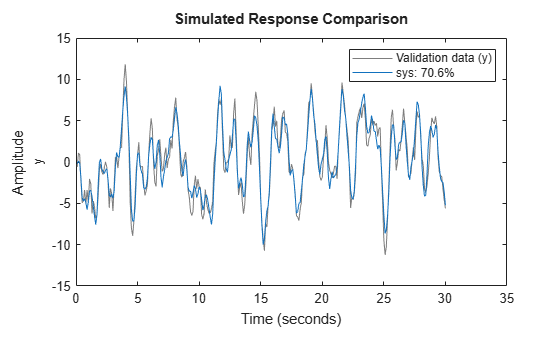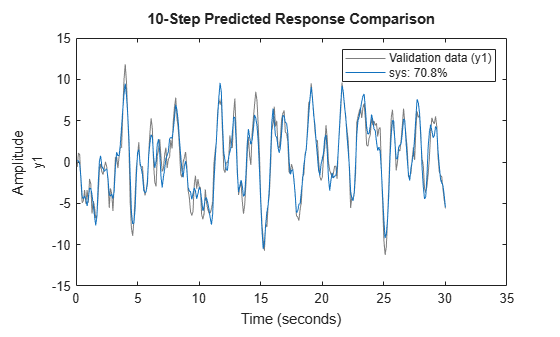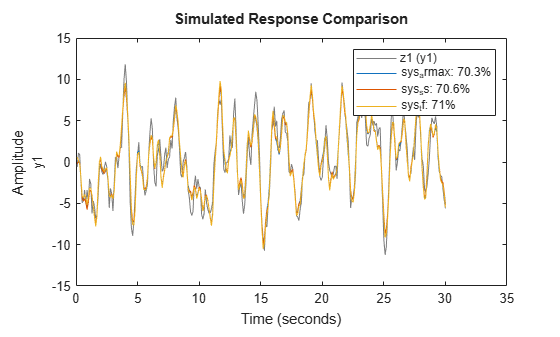Documentation

# compare

Compare identified model output and measured output

## Syntax

``compare(data,sys)``
``compare(data,sys,kstep)``
``compare(data,sys,LineSpec,kstep)``
``compare(data,sys1,...,sysN,kstep)``
``compare(data,sys1,LineSpec1,...,sysN,LineSpecN,kstep)``
``compare(___,opt)``
``````[y,fit,x0] = compare(___)``````

## Description

### Plot Results

example

````compare(data,sys)` simulates the response of a dynamic system model, and superimposes that response over plotted measurement data. The plot also displays the normalized root mean square (NRMSE) measure of the goodness of the fit between simulated response and measurement data. Use this function when you want to evaluate a set of candidate models identified from the same measurement data, or when you want to validate a model you have selected. You can use `compare` with time-domain or frequency-domain models and data.```

example

````compare(data,sys,kstep)` also predicts the response of `sys`, using a prediction horizon specified by `kstep`. Prediction uses output measurements as well as input measurements to project a future response. `kstep` represents the number of time samples between the timepoint of each output measurement and the timepoint of the resulting predicted response. For more information on prediction, see Simulate and Predict Identified Model Output.```
````compare(data,sys,LineSpec,kstep)` also specifies the line type, marker symbol, and color for the model response.```

example

````compare(data,sys1,...,sysN,kstep)` compares the responses of multiple dynamic systems on the same axes. `compare` automatically chooses the line specifications.```

example

````compare(data,sys1,LineSpec1,...,sysN,LineSpecN,kstep)` also compares the responses of multiple systems on the same axes using the line type, marker symbol, and color specified for each system.```

example

````compare(___,opt)` configures the comparison using an option set. Options include initial condition handling, data offsets, and data selection. You can use this syntax with any of the previous input-argument combinations.```

### Return Results

``````[y,fit,x0] = compare(___)``` returns the model response, `y`, goodness of fit value, `fit`, and the initial states, `x0`. No plot is generated. You can use this syntax with any of the previous input-argument combinations. However, line specifications are ignored, because there is no plotting.```

## Examples

collapse all

Identify a linear model and visualize the simulated model response with the data from which it was generated.

Load input/output measurements `z1`, and identify a third-order state-space model `sys`.

```load iddata1 z1; sys = ssest(z1,3);```

`sys `is a continuous-time identified state-space (`idss`) model.

Use `compare` to simulate the `sys` response and plot it alongside the data `z1`.

```figure compare(z1,sys)```The plot illustrates the differences between the model response and the original data. The percentage shown in the legend is the NRMSE fitness value. It represents how close the predicted model output is to the data.

To change display options in the plot, right-click the plot to access the context menu. For example:

• To plot the error between the predicted output and measured output, select Error Plot.

• To view the confidence region for the simulated response, select Characteristics -> ConfidenceRegion.

• To specify number of standard deviations to plot, double-click the plot and open the Property Editor dialog box. In the Options tab, specify the number of standard deviations in Confidence Region for Identified Models. The default value is `1` standard deviation.

Identify a linear model and visualize the predicted model response with the data from which it was computed.

Identify a third-order state-space model using the input/output measurements in `z1`.

```load iddata1 z1; sys = ssest(z1,3);```

`sys `is a continuous-time identified state-space (`idss`) model.

Now use `compare` to plot the predicted response. Prediction differs from simulation in that it uses both measured input and measured output when computing the system response. The prediction horizon defines how far in the future to predict, relative to your current measured output point. For this example, set the prediction horizon `kstep` to 10 steps, and use `compare` to plot the predicted response against the original measurement data.

```kstep = 10; compare(z1,sys,kstep)```In this plot, each `sys` data point represents the predicted output associated with output measurement data that was taken at least 10 steps earlier. For instance, the point at t = 15s is based on output measurements taken at or prior to t = 5s. The calculation of this t = 15s `sys` data point also uses input measurements up to t = 15s, just as a simulation would.

The plot illustrates the differences between the model response and the original data. The percentage shown in the legend is the NRMSE fitness value. It represents how closely the predicted model output matches the data.

To change display and simulation options in the plot, right-click the plot to access the context menu. For example, to plot the error between the predicted output and measured output, select Error Plot from the context menu. To change the prediction horizon value, or to toggle between simulation and prediction, select Prediction Horizon from the context menu.

Identify several model types for the same data, and compare the results to see which best fits the data.

Load the data, which contains `iddata` object `z1` with single input and output.

`load iddata1;`

From `z1`, identify a model for each of the following linear forms:

• ARMAX (`idpoly`) of orders 2, 3, and 1, with dead time of 0

• State space (`idss`) with three states

• Transfer function (`idtf`) with three poles

```sys_armax = armax(z1,[2 3 1 0]); sys_ss = ssest(z1,3); sys_tf = tfest(z1,3);```

Using `compare`, plot the simulated responses for the three models with `z1`.

`compare(z1,sys_armax,sys_ss,sys_tf)`For this set of data, along with the default settings for all the models, the transfer-function form has the best NRMSE fit. However, the fits for all models are within about 1% of each other.

You can interactively control which model responses are displayed in the plot by right-clicking on the plot and hovering over Systems.

Compare the outputs of multiple estimated models of differing types to measured frequency-domain data.

For this example, estimate a process model and an output-error polynomial from frequency response data.

```load demofr % frequency response data zfr = AMP.*exp(1i*PHA*pi/180); Ts = 0.1; data = idfrd(zfr,W,Ts); sys1 = procest(data,'P2UDZ'); sys2 = oe(data,[2 2 1]);```

`sys1`, an `idproc` model, is a continuous-time process model. `sys2`, an `idpoly` model, is a discrete-time output-error model.

Compare the frequency response of the estimated models to data.

`compare(data,sys1,'g',sys2,'r');`The two models have NRMSE fit values that are nearly equal with respect to the data from which they were calculated.

Modify default behavior when you compare an estimated model to measured data.

Estimate a transfer function for measured data.

```load iddata1 z1; sys = tfest(z1,3);```

`sys `is a continuous-time identified transfer function (`idtf`) model.

Suppose you want your initial conditions to be zero. The default for `compare` is to estimate initial conditions from the data.

Create an option set to specify the initial condition handling. To use zero for initial conditions, specify `'z'` for the `'InitialCondition'` option.

`opt = compareOptions('InitialCondition','z');`

Compare the estimated transfer function model output to the measured data using the comparison option set.

`compare(z1,sys,opt)`## Input Arguments

collapse all

Validation data, specified as an `iddata`, `idfrd`, or `frd` object.

If `sys` is:

• An `iddata` object, then `data` must be an `iddata` object with matching domain, number of experiments and time or frequency vectors

• A frequency-response data (FRD) model (defined as either `idfrd` or `frd`), then `data` must also be FRD

• A parametric model (such as `idss`), then `data` can be `iddata` or FRD

`data` can represent either time-domain or frequency-domain data when comparing with linear models. `data` must be time-domain data when comparing with a nonlinear model.

For examples, see:

.

Identified model, specified as a dynamic system model, an `iddata` object, or a model array.

When the time or frequency units of `data` do not match the units of `sys`, `compare` rescales `sys` to match the units of `data`.

Prediction horizon, specified as one of the following:

• `Inf` — Compare simulated response of `sys` to `data`.

• Positive finite integer — Compare predicted response of `sys` to `data`, where each predicted response point is based not only on measured input data up to that timepoint, but also on measured output data up to `kstep` timepoints earlier.

`compare` ignores `kstep` when `sys` is an `iddata` object, an FRD model, or a dynamic system with no noise component. `compare` also ignores `kstep` when using frequency response validation data.

If you specify `kstep` that is greater than the number of data samples, `compare` sets `kstep` to `Inf` and provides a warning message.

For more information on simulation and prediction, see Simulate and Predict Identified Model Output.

For an example, see Compare Predicted Response of Identified Time-Domain Model to Measured Data.

Line style, marker, and color of both the line and marker, specified as a character vector, such as `'b'` or `'b+:'`.

For more information about configuring `LineSpec`, see the `Linespec` input argument of `plot`. For an example, see Compare Multiple Estimated Models to Measured Frequency-Domain Data.

Comparison options, specified as an option set you create using `compareOptions`.

Available options include:

• Handling of initial conditions

• Sample range for computing fit numbers

• Data offsets

• Output weighting

For examples, see:

## Output Arguments

collapse all

Model response, returned as an `iddata` object, an `idfrd` object, a cell array, or an array. The output depends on the models and data you provide, as follows:

• For a single model and single-experiment data set, `y` is an `iddata` object or `idfrd` object

• For multimodel comparisons, `y` is a cell array with one `iddata` or `idfrd` object entry for each input model

• For multiexperiment data, `y` is a cell array with one entry for each experiment

• For multimodel comparisons using multiexperiment data, `y` is an Nsys-by-Nexp cell array, where Nsys is the number of models, and Nexp is the number of experiments

• If `sys` is a model array, `y` is an array with an element corresponding to each model in `sys` and experiment in `data`. For more information on model arrays, see `stack`

If `kstep` is not specified or is `Inf`, then `compare` returns the simulated response in `y`.

Otherwise, `compare` returns the predicted response. Measured output values in `data` up to time tn-`kstep` are used to predict the output of `sys` at time tn. For more information on simulation and prediction, see Simulate and Predict Identified Model Output.

The `compare` response computation requires specification of initial condition handling. By default, `compare` estimates the initial conditions to maximize the fit to data. See `compareOptions` for more information on how `compare` determines the initial conditions to use.

NRMSE fitness value indicator of how well the simulated or predicted model response matches the measurement data, returned as a vector, a matrix, or a cell array. The output depends on the models and data you provide, as follows:

• If `data` is an `iddata` object, `fit` is a vector of length Ny, where Ny is the number of outputs

• If `data` is an FRD model, `fit` is an Ny-by-Nu matrix, where Nu is the number of inputs in `data`

• For a single model and single-experiment data set, `fit` is a vector or matrix

• For multimodel comparisons, `fit` is a cell array with one entry for each input model

• For multiexperiment data, `fit` is a cell array with one entry for each experiment

• For multimodel comparisons using multiexperiment data, `fit` is an Nsys-by-Nexp cell array, where Nsys is the number of models, and Nexp is the number of experiments

• If `sys` is a model array, `fit` is an array with an element corresponding to each model in `sys` and experiment in `data`

`compare` calculates` fit` (in percentage) using:

`$\text{fit}=100\left(1-\frac{||y-\stackrel{^}{y}||}{||y-\text{mean}\left(y\right)||}\right),$`

where y is the validation data output and $\stackrel{^}{y}$ is the output of `sys`.

For FRD models — `compare` calculates `fit` by comparing the complex frequency response. The fits of the magnitude and phase curves shown in the `compare` plot are not computed by `compare` separately.

Initial conditions used to compute system response, returned as an empty array, a vector, or a cell array. The output depends on the models and data you provide, as follows:

• When `sys` is an `frd` or `iddata` object, `x0` is the empty array [ ], because initial conditions cannot be used with these objects

• For a single model and single-experiment data set, `x0` is a vector

• For multimodel comparisons, `x0` is a cell array, with one vector or matrix entry for each input model

• For multiexperiment data, `x0` is a cell array, with one entry for each experiment

• For multimodel comparisons using multiexperiment data, `x0` is an Nsys-by-Nexp cell array, where Nsys is the number of models, and Nexp is the number of experiments

• If `sys` is a model array, `x0` is an array with an element corresponding to each model in `sys` and experiment in `data`

By default, `compare` uses `findstates` to estimate `x0`. To change this behavior, set the `'InitialCondition'` option in `opt` (see `compareOptions`). If you have input/output history that immediately precedes your start point, you can set `'InitialCondition'` to that history data. `compare` then uses `data2state` to compute the end state of the history data, and thus the start state for the simulation. Other choices include setting initial conditions to zero, or to specific values that you have determined previously. For more information about finding initial conditions, see Estimate Initial Conditions for Simulating Identified Models.

If you are using an estimation model that does not explicitly use states, `compare` first converts the model to its state-space representation and then maps the data to states. For more information, see `compareOptions`.

## Tips

• The NRMSE fit result you obtain with `compare` may not precisely match the fit value reported in model identification. These differences typically arise from mismatches in initial conditions, and in the differences in the prediction horizon defaults for identification and for validation. The differences are generally small, and should not impact your model selection and validation workflow. For more information, see Resolve Fit Value Differences Between Model Identification and compare Command.

• `compare` matches the input/output channels in `data` and `sys` based on the channel names. Thus, it is possible to evaluate models that do not use all the input channels that are available in `data`. This flexibility allows you to compare multiple models which were each identified independently from different sets of input/output channels.

• The `compare` plot allows you to vary key parameters. For example, you can interactively control:

• Whether you generate a simulated or predicted response

• Prediction horizon value

• Initial condition handling

• Which experiment data you view

• Which system models you view

To access the controls, right-click the plot to bring up the options menu.

#### Introduced in R2006a

##### SupportGet trial now